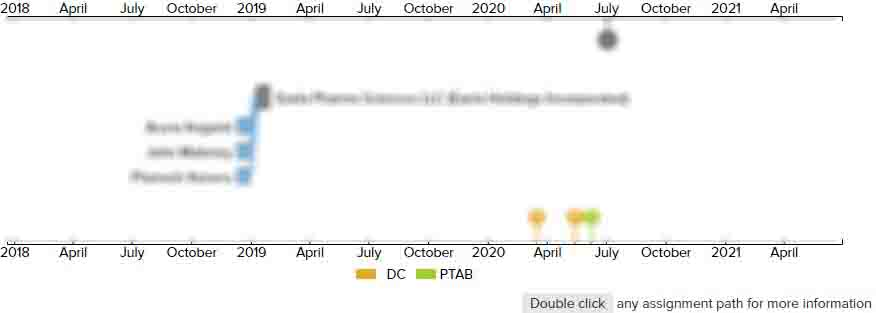# Methods for computing rolling analyte measurement values, microprocessors comprising programming to control performance of the methods, and analyte monitoring devices employing the methods

• US 7,011,630 B2
• Filed: 06/21/2002
• Issued: 03/14/2006
• Est. Priority Date: 06/22/2001
• Status: Active Grant
##### First Claim
Patent Images

1. A method of increasing the number of analyte measurement values related to the amount or concentration of an analyte in a subject as measured using an analyte monitoring device, said method comprisingproviding a series of analyte-related signals obtained from the analyte monitoring device over time, wherein (a) two or more contiguous analyte-related signals are used to obtain a single analyte measurement value (M), (b) analyte-related signals are not used to calculate more than one analyte measurement value, and (c) said two or more contiguous analyte-related signals, used to obtain the single analyte measurement value, comprise first and last analyte-related signals of the series;

• mathematically computing rolling analyte measurement values, wherein (i) each rolling analyte measurement value is calculated based on two or more contiguous analyte-related signals from the series of analyte-related signals obtained from the analyte monitoring device, (ii) a subsequent rolling analyte measurement value is mathematically computed by dropping said first analyte-related signal and including an analyte-related signal contiguous and subsequent to the last analyte-related signal, (iii) further rolling analyte measurement values are obtained by repeating the dropping of the first analyte-related signal used to calculate the previous rolling analyte measurement and including an analyte-related signal contiguous and subsequent to the last analyte-related signal used to calculate the previous rolling analyte measurement, and (iv) each rolling analyte measurement value provides a measurement related to the amount or concentration of analyte in the subject; and

increasing the number of analyte measurement values derived from the analyte-related signals in the series of analyte-related signals obtained from the analyte monitoring device by serially calculating rolling analyte measurement values, thereby increasing the number of analyte measurement values relative to the number of analyte measurement values provided when two or more contiguous analyte-related signals are used to obtain a single analyte measurement value (M) and analyte-related signals are not used to calculate more than one analyte measurement value.

• ##### 4 Assignments
Timeline View
Assignment View×